Next: Extension to Oblique Incidence Up: Wave Propagation in Inhomogeneous Previous: WKB Approximation

# Reflection Coefficient

Consider an ionosphere in which the refractive index is a slowly varying function of height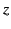above the surface of the Earth. Let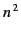be positive for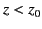, and negative for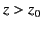. Suppose that an upgoing radio wave of amplitude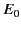is generated at ground level (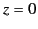). The complex amplitude of the wave in the region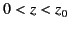is given by the upgoing WKB solution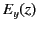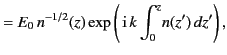(1080)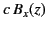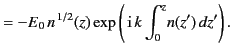(1081)

The upgoing energy flux is given by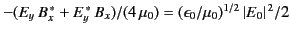. In the region, the WKB solution takes the form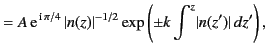(1082)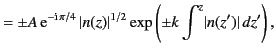(1083)

whereis a constant. These solutions correspond to exponentially growing or decaying waves. Note that the magnetic components of the waves are in phase quadrature with the electric components. This implies that the Poynting fluxes of the waves are zero: in other words„ the waves do not transmit energy. Thus, there is a non-zero incident energy flux in the region, and zero energy flux in the region. Clearly, the incident wave is either absorbed or reflected in the vicinity of the plane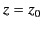(where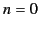). In fact, as we shall prove later, the wave is reflected. The complex amplitude of the reflected wave in the regionis given by the downgoing WKB solution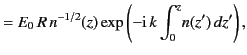(1084)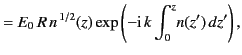(1085)

where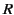is the coefficient of reflection. Suppose, for the sake of argument, that the planeacts like a perfect conductor, so that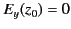. It follows that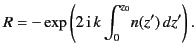(1086)

In fact, as we shall prove later, the correct answer is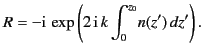(1087)

Thus, there is only a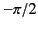phase shift at the reflection point, instead of the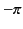phase shift that would be obtained if the planeacted like a perfect conductor.Next: Extension to Oblique Incidence Up: Wave Propagation in Inhomogeneous Previous: WKB Approximation
Richard Fitzpatrick 2014-06-27